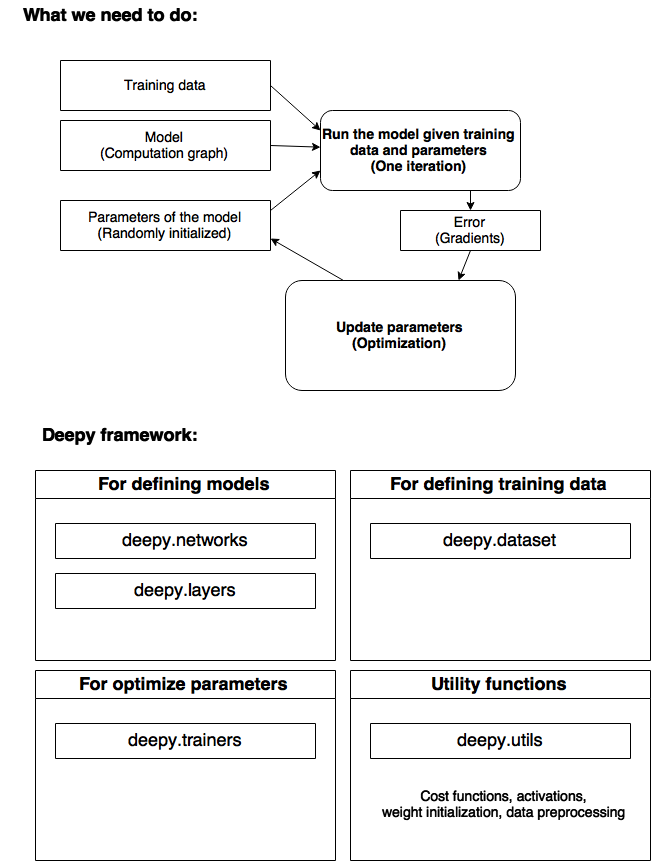# Welcome to deepy

deepy is a deep learning framework for designing models with complex architectures.

Many important components such as LSTM and Batch Normalization are implemented inside.

Although highly flexible, deepy maintains a clean high-level interface.

# Get started in 30 seconds

``````\$ git clone https://github.com/zomux/deepy
\$ cd deepy
\$ pip install -r requirements.txt
\$ source bin/cpu_env.sh
\$ python experiments/mnist/mlp_dropout.py
``````

## How to design your first simple neural network

First make a new python file for defining your first model.

``````\$ mkdir my_experiments
\$ touch my_experiments/first_model.py
``````

Edit this file, perhaps with vim.

``````\$ vim my_experiments/first_model.py
``````

From now on, you are going to write python codes for defining a neural network.

First import everything from deepy.

``````from deepy import *
``````

Suppose you want to design a multi-layer feed-forward network to classify MNIST numbers.

Then you have four questions to consider:

• What cost function to use
• What is the architecture of the network
• What optimization method to use

With deepy you can implement a network easily and intuitive once you got the answers.

Here, we give a simple design of a feed-forward neural network.

``````model = NeuralClassifier(input_dim=28 * 28)

model.stack(
Dense(256, 'relu'),
Dense(256, 'relu'),
Dense(10, 'linear'),
Softmax()
)

trainer.run(MiniBatches(MnistDataset(), batch_size=20))
``````

Now you are done, simple run the following command to train your first model.

``````\$ python my_experiments/first_model.py
``````

``````model.save_params("my_experiments/my_first_model.gz")